SPSS TUTORIALS BASICS ANOVA REGRESSION FACTOR CORRELATION

# Median – Simple Tutorial & Examples

The median is the middle value after sorting all values for an odd number of values. For an even number of values, it's the average of the 2 middle values after sorting all values. The examples below from this Googlesheet (read only) will make this perfectly clear.

## Median - Simple Data Examples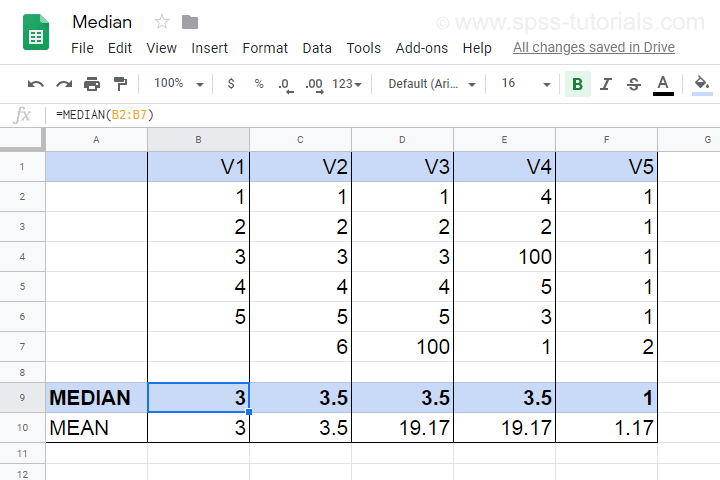• V1 holds values 1 through 5 sorted ascendingly. The median -middle value- is 3.
• V2 holds values 1 through 6 sorted ascendingly. The median is 3.5. It is the average of the 2 middle values 3 and 4.
• V3 is V2 with 6 replaced by 100. This greatly affects the mean but the 2 middle values -and hence the median- stay the same.
• V4 holds the values of V3 in random order. The median is not the average of the 2 middle values unless we first sort them.
• V5 contains ties: the value 1 occurs 5 times. Since the values are sorted, the median is the average of the 2 middle values (1 and 1).

Note that for V2 through V4, the median is the value that separates
the 50% highest values from the 50% lowest values.
This turns out to hold for most (semi)continuous variables that we find in real-world data such as

• exact monthly incomes in dollars,
• body weight in grams or
• age in days.

However, it may not hold at all for heavily tied data (such as V5) or small numbers of observations.

## Relation Median and Mean

We'll discuss the pros and cons of medians versus means in a minute. Let's first see how they relate in the first place. This depends mostly on the skewness of the frequency distribution of some variable: the median is equal to the mean
for symmetrically distributed variables
which implies skewness = 0. The histogram shown below illustrates this point.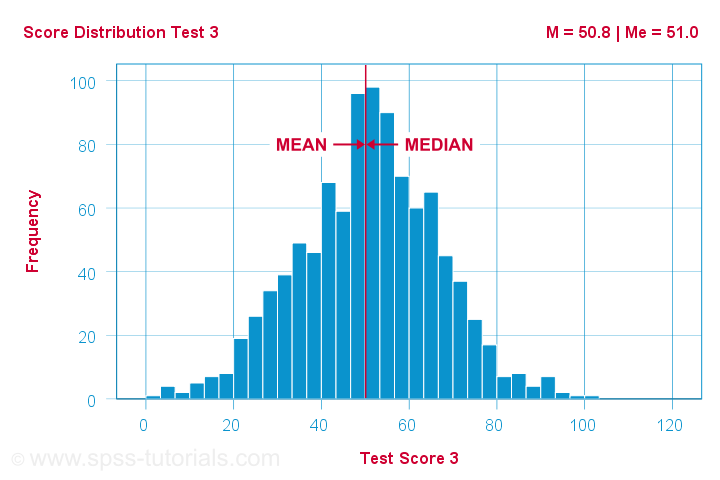Skewness is basically zero for these 1,000 test scores. The sample mean (M) = 50.8 while the median (Me) = 51.0. The red lines indicating them on the x-axis are indistinguishable.
Different patterns occur when skewness is substantial. First off, the median is smaller than the mean
for positively skewed variables
as shown below.What basically happens here is that some very high scores affect the mean but not the median. We already saw this in our initial examples: changing {1,2,3,4,5,6} to {1,2,3,4,5,100} greatly affects the mean but the median is 3.5 for both variables. The histogram above shows the exact same phenomenon but it uses more realistic data.
As you can probably guess by now, the opposite also holds: the median is larger than the mean
for negatively skewed variables
as illustrated by the histogram below.What basically happens here is that the very low scores “drag down” the mean. The median, however, is unaffected by these.

## Median - Strengths & Weaknesses

Thus far, this introduction implicitly pointed out some strengths of the median compared to the mean:

• The median is not sensitive to outliers. So perhaps the mean salary for some people is high due to a single billionaire. I'd rather know the median salary in this case. This'll tell me (roughly) which salary separates the 50% lowest from the 50% highest incomes. It's a more realistic estimate of what these people tend to earn.
• Means are applicable only to quantitative variables. Medians are also suitable for ordinal variables. However, ordinal variables typically have tons of ties (values that occur more than once). For such variables, medians may be misleading as shown below.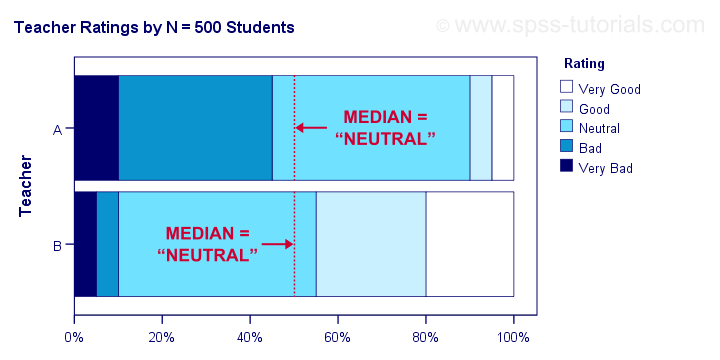Although teacher B is rated much more favorably than teacher A, their median ratings are identical.

Apart from these strengths, medians have some weaknesses too:

• Medians are unsuitable for numeric calculations. For instance, sums can be calculated from means and sample sizes but not from medians. The difference between 2 means is easily interpretable but the difference between 2 medians hardly so.
• In the presence of ties, very different variables may have similar medians.
• The median may not actually exist. For instance, if two people have 0 and 1 children, their median is 0.5 children.
• The median is said to fluctuate more from sample to sample than the mean. That is, it is less stable and has a larger standard error.

Finding medians is super easy with Googlesheets. For example, typing =MEDIAN(B2:B7) into any cell results in the median of cells B2 through B7 (assuming all non empty cells contain numbers). Some more examples are shown in this Googlesheet (read only).

## Finding Medians in SPSS

In SPSS, the best option to find medians is from AnalyzeCompare MeansMeans
Use this dialog to create a table showing a wide variety of descriptive statistics including the mean, standard deviation, skewness, kurtosis and more. Optionally, these are reported for separate groups defined by “Independent List”.An even faster option is typing and running the resulting syntax -a simple MEANS command- such as means v1 to v5
/cells count mean median.
An example of the resulting table -after some adjustments- is shown below.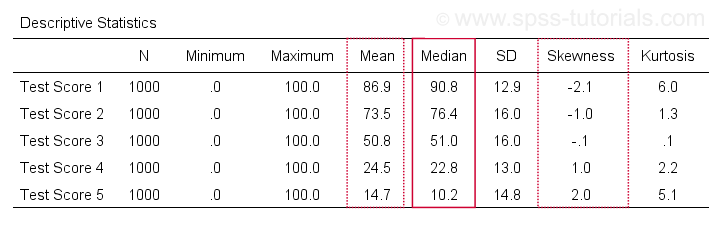Notice the huge positive correlation between skewness and (mean - median): the median is larger than the mean insofar as a variable is more negatively (left) skewed. The opposite pattern -mean larger than median- occurs for positively (right) skewed variables. This was previously illustrated with some histograms based on the same data file as this table.

## Statistical Significance for Medians - Sign Tests

Among the most popular statistical techniques are t-tests. These test if the difference between 2 means is statistically significant. But what if we want to test for medians instead of means? In this case we'll end up with one of 3 median tests, sometimes called sign tests:

A sign test for 1 median basically works like this:

• each value smaller than the hypothesized median is replaced by a minus (-) sign;
• values larger than the hypothesized median are replaced by plus (+) signs;
• if the hypothesized median is correct, then some 50% of all signs should be plusses;
• a binomial test examines if the sample proportion of plusses is significantly different from 0.5.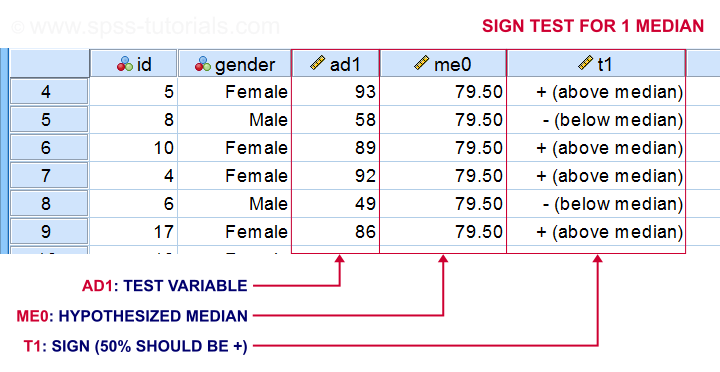The other sign tests follow the same basic reasoning. Sign tests are not very popular because ties are problematic for them and they tend to have low statistical power.

# Tell us what you think!

*Required field. Your comment will show up after approval from a moderator.

# THIS TUTORIAL HAS 14 COMMENTS:

•### By Mohammad Zaheer on January 11th, 2021

I need all spss materials

•### By Pam Bartels on February 11th, 2021

Great information to help guide my research

•### By Mikael on June 3rd, 2021

After I read this I still don't know how SPSS Modeler finds value for Median.

I tested with a fake dataset of 40 observations 20th being 4 and 21st 5. Modeler gives me Median of 4,600. Isn't this incorrect as it ought to be 4,5000?

•### By Ruben Geert van den Berg on June 4th, 2021

Hi Mikael!

First off, all our tutorials are about SPSS statistics, SPSS not modeler.

Anyway, your observations sound weird as it should be 4.5 indeed if those values are really 4.000... and 5.000.... and there's really 40 (unweighted, unfiltered) observations.

Possible causes could be:

-a WEIGHT variable being in effect
-a FILTER variable being in effect
-hidden decimal places in your numeric values

Anyway, try and replicate the analysis in Excel or some other alternative and see what comes up.

Whenever I think SPSS is giving wrong results, it usually turns out to be me who's wrong unless it's about highly specialized situations or topics.

Hope that helps!

SPSS tutorials